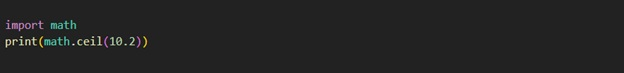# Python Math Ceil Method

Python Math Ceil

Now, we are going to learn about the ceil method. As the name of the method says, this method returns the ceiling of the given argument number. This simply means that we are going to get the smallest integer, greater than or equal to the given number argument.

Let’s have a look at a simple example, which tries to demonstrate the same thing.## Python Math Ceil

As you can see in the above program, we have imported the math class, since it is a module, we need to import it, in order to use it in our programs. Also, with the import statement from the above program, we can understand how we can write the import statement, to import some modules into our program.

After that, we are calling the ceil method, and notice how we are calling the ceil method. We are just doing math.ceil, and then passing the argument number. We get the smallest integer, greater than or equal to the given number.   If you try to run the above program, it would print 11 as the output, since 11 is the smallest integer greater than or equal to 10.2.

So, as and when required, we can make use of the ceil method from the math module. Remember that the ceil method returns the smallest integer greater than or equal to the given argument number.